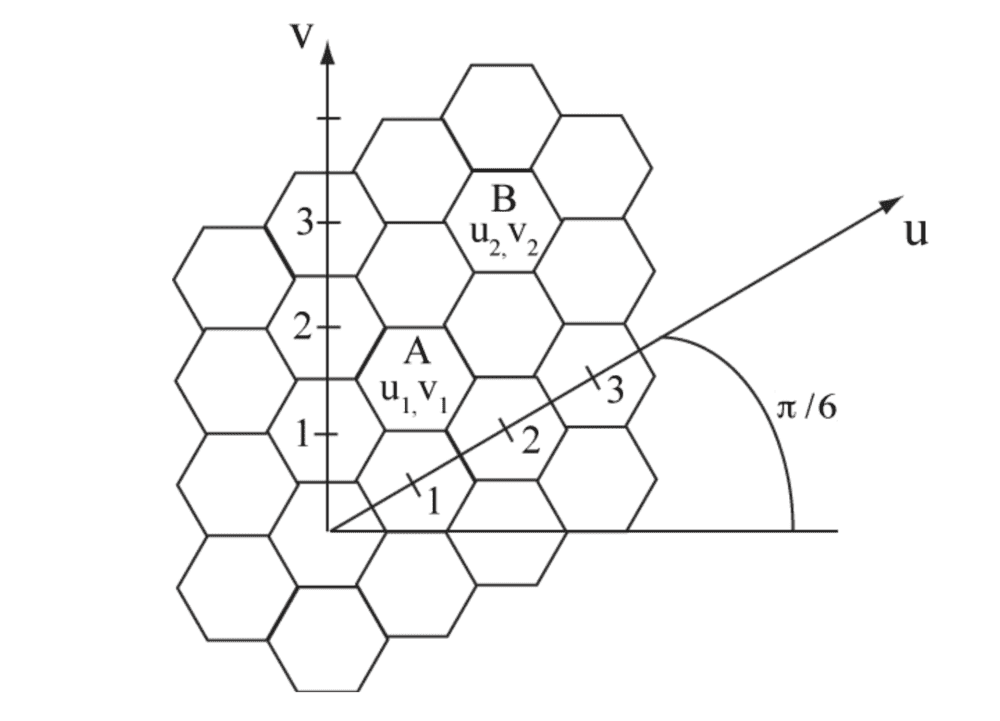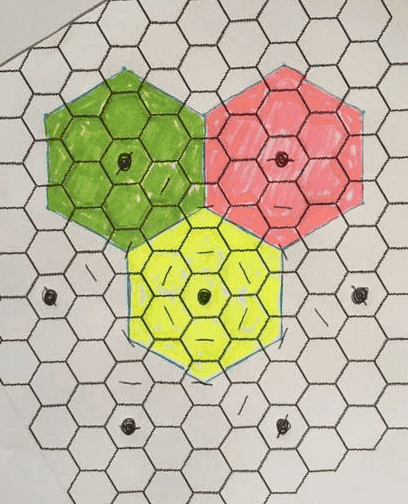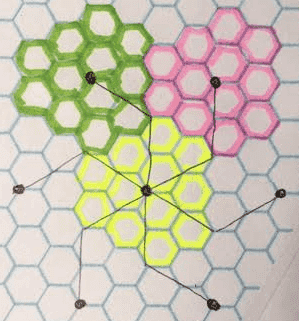# Mobile Comms: Number of Cells in Hexagonal Pattern

• Engineering
Master1022
Homework Statement:
Why is the number of cells in the radius ## D ## determined by ## N_c = D_R^2 ## where ## D_R = \sqrt{i^2 + j^2 + ij} ##
Relevant Equations:
area
Hi,

I was looking at some notes and trying to understand the following statement which refers to the diagram below.
"The number of cells in the radius ## D ## determined by ## N_c = D_R^2 ## where ## D_R = \sqrt{i^2 + j^2 + ij} ##
where ## i## and ##j## are the number of cells along the ## u ## and ## v ## axes respectively.From what I understand, B is at (2, 2) in (u, v) coordinates and radius ##D## is ## = \sqrt{2^2 + 2^2 + (2)(2)} \cdot R \sqrt{3} ##. Therefore, the number of cells within the radius ## D ## should be ## 12 ##. I cannot see how this is the case, no matter how I try to encircle cells...

I think I am missing something quite simple. Any help would be greatly appreciated.

Homework Helper
Gold Member
I think that the formula Nc = i2 +j2 +ij refers to the number of hexagonal cells in a "cluster", where clusters are distanced by i and j.

For i=2, j=2, this results in clusters that share cells, fractionally. Add the fractional cells to the non-shared cells and you will get 12.

Last edited:
•Master1022
Master1022

I think that the formula Nc = i2 +j2 +ij refers to the number of hexagonal cells in a "cluster", where clusters are distanced by i and j.

For i=2, j=2, this results in clusters that share cells, fractionally. Add the fractional cells to the non-shared cells and you will get 12.

I am struggling to picture this on the image... Is there any chance you could edit/add something to the image posted to show what you are saying? I will keep trying to think about it in the meantime.

Homework Helper
Gold Member
Perhaps if you study this image you will see what I mean by fractional cells. The black dots are distanced by i=2, j=2. [edited to match your original orientation]•Master1022
Homework Helper
Gold Member
FWIW, with a change of grid origin, you can get a different cluster pattern that eliminates the fractional cells:Last edited:
•Master1022
Master1022
Thank you very much @lewando for taking the time to produce those pictures! They are extremely helpful!

•lewando